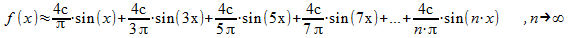# Part 3 - Wavetables

This part 3 of the tutorial shows the basic usage of the wavetable functionality which is implemented in the . You can use this wavetable functionality to customize the waveform of an oscillator. The subject of this part 3 is rebuilding that standard square type (`oscillator.type="square";`) of the built-in oscillator with the wavetable functionality.
In general if you want to use such wavetable functionality to rebuild a certain waveform (like sinus, sawtooth, ...) you need the representation of this waveform. Getting such a representation out of an arbitrary periodical function requires a mathematical process called Fourier analysis. This analysis itself is not part of this tutorial.
The following formula represents the square waveform as Fourier series:Or in other words: A Fourier series aproximates a waveform (here the "square curve") with a sum of cosine an sine terms. In this special case the cosine and the even sine parts cancel out (they become zero) as solution of the Fourier analysis of the square waveform.
So only the odd sine parts are not zero and must be calculated (for this special waveform).
In general cosine and sine parts could remain as result of the Fourier analysis.
To use that in conjunction with the createPeriodicWave-method of the Web Audio API two arrays () must be prepared. Additionally the variable n holds the number of series for the formula. Mathematically this n should go to infinity to exactly match the waveform with the Fourier series. It's obvious that n cannot be infinite in a real program. But you can say in terms of approximation the higher the value of n is the better accuracy to the waveform is achieved. The maximum size that the createPeriodicWave-method allows (according to the Web Audio API spec) is 4096.

``` var n = 4096; var real = new Float32Array(n); var imag = new Float32Array(n); ```
The array named real reserves the memory for the cosine coefficients of the Fourier series. The sine coefficients are saved in the array named imag. Keep in mind that both arrays are initialised with zeros this way. So only the non zero parts of the sine terms must be calculated and placed at the right positions in the imag array for this example.

``` for(var x = 1; x < n; x+=2) {     imag[x] = 4.0 / (Math.PI*x); } ```
This for-loop leaves the first value of the imag-array as zero because x starts with 1. If you put x=0 in the formula above you see that the solution is zero. With x+=2 the loop jumps over every even number so only the odd indexed imag[x]-values are calculated. The createPeriodicWave-method does the adding of the sine parts automatically. Additionally it "normalizes" the calculation so the constant c can be left out by being 1. This reduces the code for imag[x] as can be seen in the code above.
Given an audio context named context:

``` var context = new AudioContext(); ```
the createPeriodicWave-method can now be called the following way:

``` var wt = context.createPeriodicWave(real, imag); ```
The created wavetable wt is now given to an oscillator via the setPeriodicWave-method:

``` var oscillator = context.createOscillator(); oscillator.setPeriodicWave(wt); oscillator.start(0); ```
Finally the whole JavaScript code of the example:

``` var context = new AudioContext(); var oscillator = context.createOscillator(); var n = 4096; var real = new Float32Array(n); var imag = new Float32Array(n); for (var x = 1; x < n; x+=2) {     imag[x] = 4.0 / (Math.PI*x); } var wt = context.createPeriodicWave(real, imag); oscillator.setPeriodicWave(wt); oscillator.noteOn(0); var volumeNode = context.createGain(); volumeNode.gain.value = 0.5; oscillator.connect(volumeNode); function startTone() {     volumeNode.connect(context.destination); } function stopTone() {     volumeNode.disconnect(); } function changeFrequency(value) {     oscillator.frequency.value = value; } function changeVolume(value) {     volumeNode.gain.value = value; } ```
Now the oscillator should sound like the standard type square (`oscillator.type="square";`). If you play around with n you can figure out how much accuracy you need for such a waveform.

Frequency: 440.0 Hz
Volume: 0.5

If you don't want to calculate wavetables yourself you can also use them in precalculated form and put these in the createWaveTable method. Often these wavetables are downloadable as format.